DNA UNIT QUIZ/2018 Take Home
Last name *
period *
1. 3' ATC AGC GCT GGC GCC 5' ....Is a sequence of DNA that is part of a gene. If this piece of DNA were to be transcribed, how many codons would be present?
2. One human disease is caused by a substitution in one base in a gene from GAA to GUA. This disease is the result of a
3. Which of the following best describes the composition of a nucleotide?
4. An enzyme moves along a strand of DNA and produces a new nucleic acid strand. Part of the new strand is shown below. GTACTCGGCAATTTCGCA Which of the following cellular processes created the new strand?
Which of the following is a characteristic of RNA but not DNA?
6. Diagram 1 below, what process is being illustrated?
Diagram 1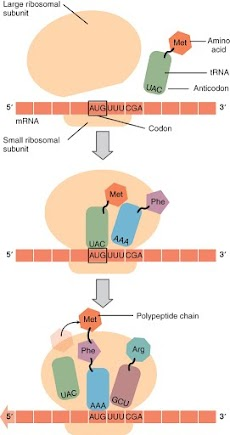7. Refer to diagram 2, below. Which of the following is NOT visible or present?
Diagram 2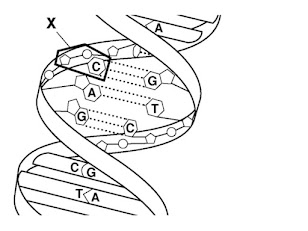8. Refer to diagram 2 above. If you unzipped and replicated the above diagram, what would you need to "break" first?
10. Using the diagram below (diagram 3), what portion of the protein molecule is coded for the the following mRNA? mRNA = AGU AAG UGC UUC
Diagram 3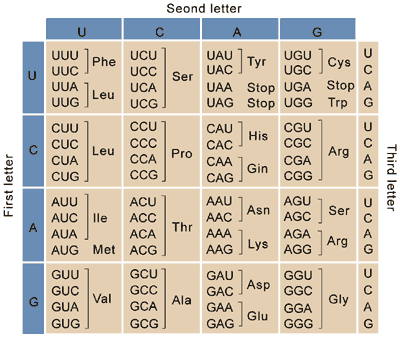11. Refer to the above diagram (diagram 3). Suppose that you are given a protein containing the following sequence of amino acids: arginine (arg)-Methionine (met)- aspartic acid (asp). Which DNA sequence would have coded for this amino acid sequence?
12. Which of the following represents the codons that correspond to this segment of DNA: TATCAGGAT?
Diagram 4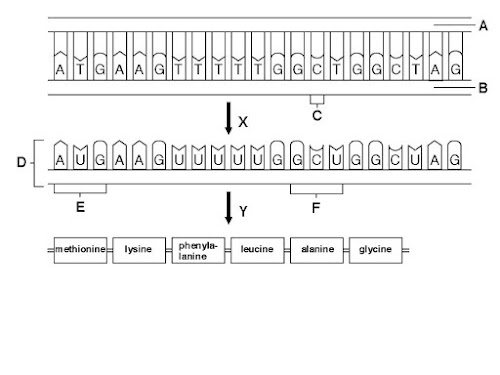13. Use diagram 4, above to answer. Letter Y is a process called
14. How many codons are needed to specify three amino acids
15. Which of the following base pair sequences could be produced in DNA replication Given the following DNA strand: 3' TCA GTA 5'
16. Which of the following correctly ranks the structures in order of size, from SMALLEST to LARGEST?
17. The nucleotide sequence of DNA is TTA. A messenger RNA molecule with a complementary codon is transcribed from the DNA. In the process of protein synthesis, a transfer RNA pairs with the mRNA codon. What is the base sequence of the tRNA anticodon?
18.Ultraviolet (UV) radiation is damaging because it _____.
19. The information in DNA is contained in _____.
20. A messenger RNA molecule for making a protein is made in the nucleus and sent out to a ribosome. The ribosome reads the mRNA and makes a protein containing 120 amino acids. The mRNA consisted of at least how many codons?
21. At one point as a cell carried out its day-to-day activities, the nucleotides G A T were paired with the nucleotides C U A. This pairing occurred
23. In a nucleotide, the bases are always attached to the _______________ carbon of the sugar.
24. DNA replication is called semiconservative because half of each of the two new strands of DNA is "old" DNA from the original DNA molecule and half is "new" DNA.
25. Which of the following terms is LEAST closely related to the others?
26. According to Hank (in the videos on KHAN), the molecule Titin is
27. What is meant by the description "antiparallel" regarding the strands that make up DNA?
28. In an analysis of the nucleotide composition of DNA, which of the following will be found?
29 Match the # in the diagram with the correct word or words. In diagram 5 below, #1 is pointing to
diagram 5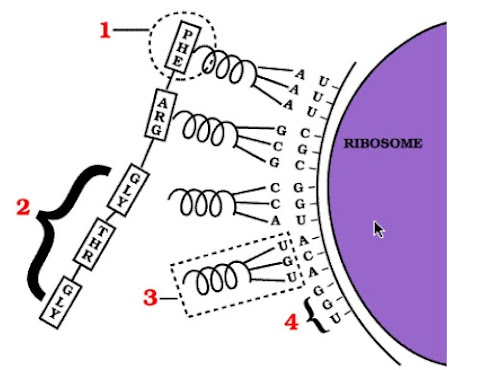30 Match the # in the diagram with the correct word or words. In diagram 5 above, #3 is pointing to